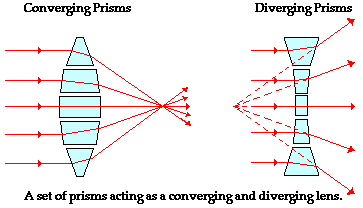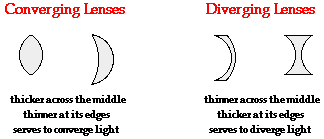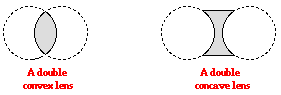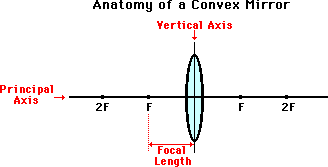Refraction and the Ray Model of Light - Lesson 5 - Image Formation by Lenses

# The Anatomy of a Lens

If a piece of glass or other transparent material takes on the appropriate shape, it is possible that parallel incident rays would either converge to a point or appear to be diverging from a point. A piece of glass that has such a shape is referred to as a lens.A lens is merely a carefully ground or molded piece of transparent material that refracts light rays in such as way as to form an image. Lenses can be thought of as a series of tiny refracting prisms, each of which refracts light to produce their own image. When these prisms act together, they produce a bright image focused at a point.

### Types of Lenses

There are a variety of types of lenses. Lenses differ from one another in terms of their shape and the materials from which they are made. Our focus will be upon lenses that are symmetrical across their horizontal axis - known as the principal axis. In this unit, we will categorize lenses as converging lenses and diverging lenses. A converging lens is a lens that converges rays of light that are traveling parallel to its principal axis. Converging lenses can be identified by their shape; they are relatively thick across their middle and thin at their upper and lower edges. A diverging lens is a lens that diverges rays of light that are traveling parallel to its principal axis. Diverging lenses can also be identified by their shape; they are relatively thin across their middle and thick at their upper and lower edges.A double convex lens is symmetrical across both its horizontal and vertical axis. Each of the lens' two faces can be thought of as originally being part of a sphere. The fact that a double convex lens is thicker across its middle is an indicator that it will converge rays of light that travel parallel to its principal axis. A double convex lens is a converging lens. A double concave lens is also symmetrical across both its horizontal and vertical axis. The two faces of a double concave lens can be thought of as originally being part of a sphere. The fact that a double concave lens is thinner across its middle is an indicator that it will diverge rays of light that travel parallel to its principal axis. A double concave lens is a diverging lens. These two types of lenses - a double convex and a double concave lens will be the only types of lenses that will be discussed in this unit of The Physics Classroom Tutorial.### The Language of Lenses

As we begin to discuss the refraction of light rays and the formation of images by these two types of lenses, we will need to use a variety of terms. Many of these terms should be familiar to you because they have already been discussed during Unit 13. If you are uncertain of the meaning of the terms, spend some time reviewing them so that their meaning is firmly internalized in your mind. They will be essential as we proceed through Lesson 5. These terms describe the various parts of a lens and include such words as

 Principal axis Vertical Plane Focal Point Focal Length

If a symmetrical lens were thought of as being a slice of a sphere, then there would be a line passing through the center of the sphere and attaching to the mirror in the exact center of the lens. This imaginary line is known as the principal axis. A lens also has an imaginary vertical axis that bisects the symmetrical lens into halves. As mentioned above, light rays incident towards either face of the lens and traveling parallel to the principal axis will either converge or diverge. If the light rays converge (as in a converging lens), then they will converge to a point. This point is known as the focal point of the converging lens. If the light rays diverge (as in a diverging lens), then the diverging rays can be traced backwards until they intersect at a point. This intersection point is known as the focal point of a diverging lens. The focal point is denoted by the letter F on the diagrams below. Note that each lens has two focal points - one on each side of the lens. Unlike mirrors, lenses can allow light to pass through either face, depending on where the incident rays are coming from. Subsequently, every lens has two possible focal points. The distance from the mirror to the focal point is known as the focal length (abbreviated by f). Technically, a lens does not have a center of curvature (at least not one that has any importance to our discussion). However a lens does have an imaginary point that we refer to as the 2F point. This is the point on the principal axis that is twice as far from the vertical axis as the focal point is.As we discuss the characteristics of images produced by converging and diverging lenses, these vocabulary terms will become increasingly important. Remember that this page is here and refer to it as often as needed.

### We Would Like to Suggest ...Why just read about it and when you could be interacting with it? Interact - that's exactly what you do when you use one of The Physics Classroom's Interactives. We would like to suggest that you combine the reading of this page with the use of our Optics Bench Interactive. You can find this in the Physics Interactives section of our website. The Optics Bench Interactive provides the learner an interactive enivronment for exploring the formation of images by lenses and mirrors. Its like having a complete optics toolkit on your screen.

Next Section: